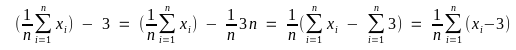# how do I modify the argument of =AVERAGE() function

How do I subtract a constant from the argument of
=AVERAGE()
function :
=AVERAGE((A2:A21)-3)
doesn’t work.

What is it you are trying todo? Subtract the const value (3) from each element in the range?

Mathematically it is the same thing to subtract 3 from the average of values or to build the average of (values - 3):but of course you could use:

 `{=AVERAGE(A2:A21-3)}` (enter `=AVERAGE(A2:A21-3)` and finalize with `CTRL+SHIFT+ENTER`, to get the array formula – or–

 `=SUMPRODUCT(AVERAGE(A2:A21-3))`

Thats one reason, why i ask what @max2 actually wants todo. I seemed a bit odd, so i suspected a
xy-problem XY problem - Wikipedia.

I wanted to compute the k-th central AND non-central moment of a given array of \$X_i\$'s for a given natural number k. I want a macro for this 2 moments. The X_i’s are A:37 to A56

@max2

I wanted to compute the k-th central AND non-central moment of a given array of \$X_i\$’

You asked that in another question here: Third central and non-central moment in LO. Don’t expect a question answered which is not asked here. This question here is: How do I subtract a constant from the argument of =AVERAGE() function and this question has been answered by @Hrbrgr.

English = Average; the same as; German = Mittelwert